# Numb3rs 113: Manhunt

In this episode, Charlie uses Bayesian inference and Markov chains to help his brother figure out the cause of a bus crash which let two prisoners escape from a prison bus.

## Bayesian Inference

Bayesian inference is the process of adjusting your belief in the probability of the truth of some statement based on new events. As a silly example, you may believe that it is extremely unlikely that there are any purple cows in the world. However, if you see a purple cow, then you would obviously change your belief to the belief that there is at least one purple cow in the world.

Bayes' rule is attributed to the Reverend Thomas Bayes, a Presbyterian minister who lived from 1701 to 1761 in a work entitled "Essay Towards Solving a Problem in the Doctrine of Chances." This was a response to a work entitled "The Doctrine of Chances" by Abraham de Moivre, one of Bayes' mathematical collegues. A link to Bayes original paper (which was published posthumously) can be found here.
To give a mathematical basis for this inference process, we need to state Bayes' theorem. Suppose we have two different events, A and B. We'll write P(A) for the probability that A occurs and P(B) for the probability that B occurs. Also, we'll write P(A|B) for the probability that A happens given that B happens. We can rewrite this as P(A|B) = P(A and B)/P(B), that is, P(A|B) is the probability that A and B both happen divided by P(B). Then Bayes rule is the following formula:This can be proved very simply using the formula for P(A|B). Now let's look at an example.

Let's say that Billy Bob has two boxes, one with one fair die in it, labelled with the numbers 1 through 6, and one with two fair dice in it, labelled with the numbers 1 through 6. Let's say he picks one box at random and then rolls the dice in the box. Let's use Bayes' rule to figure out the probability that he picked the box with one die given the fact that the total of the die (or dice) he rolled was a 3. First we need to translate the words into the symbols we used in the previous paragraph. Let's use A to denote the event that Billy Bob picks the box with one die and B the event that he rolls a 3. Then as we assumed, P(A) = .5, and we can calculate P(B) = .5*(1/6) + .5*(2/36)= 4/36 = 1/9. This is because there's a 1/2 chance he'll pick box 1, and if he does there's a 1/6 chance he'll roll a 3. Also, there's a 1/2 chance he'll pick box 2 and a 2/36 chance he'll roll a 3 (he can get a 1,2 or a 2,1). Now since what we want to figure out the probability that he picked box 1 (event A) given the fact that he rolled a 3 (event B), we want to use Bayes rule to figure out P(A|B). To use the formula we need to figure out what P(B|A) is. This is the probability that he rolls a 3 given the fact that he picks box A, so P(B|A) = 1/6. Then P(A|B) = (1/6)*(1/2)/(1/9) = 3/4. This makes sense because if he picks the box with two dice he is unlikely to get a 3, while if he picks the box with 1 die he is more likely to get a 3.

1. If A is as above and B is the event that Billy Bob rolls a 6, what is P(A|B)?
2. If A is as above and B is the event that Billy Bob rolls a 5 or a 6, what is P(A|B)?
3. If A is as above and B is the event that Billy Bob rolls a 1, what is P(A|B)?
The Monty Hall Problem: This problem is loosely based on a situation that occurred in the tv show "Let's Make a Deal." Let's say you're on a game show and the host presents you with three doors. One has a shiney new car behind it, and the other two have goats. The object (of course) is to get the car and not to get a goat. Now the game goes like this. First, you pick a door. Then at least one of the two doors you did not pick has a goat behind it. The game show host knows what the shut doors have behind them and he deliberately opens one of the two that you did not pick to show you a goat (if both have a goat, he randomly picks the one to open). Then he lets you either stay with your original choice of door or switch to the other unopened door. Then you get what is behind that door. Now the goal of the problem is to use Bayes' rule to figure out whether it is better to switch or stay put. Let's say you picked door 1 and he opened door 2. Let A be the event that door 3 has a car behind it and let B be the event that he opened door 2.
1. What is P(A)? Remember this refers to the probability that A occurs before you know anything that happens.
2. What is P(B)? Remember this refers to the probability that B occurs before you know anything that happens.
3. What is P(B|A)? This is the probability that door 3 has a goat behind it given the fact that door 2 has a goat behind it. (Hint: how many goats and cars are left after door 2 has been opened?)
4. What is P(A|B)?
5. Now should you switch?

## Markov Chains

A Markov chain is a series of random events where the outcome of event n is only dependent on the outcome of the event n-1. For example, let's say there is a number line where each integer has a dot on it, and let's say that there is also an evil but useless robot standing on position 0. The robot has a quarter, and he flips it to determine whether he should go left or right. If he gets heads, he'll move to position 1, and if he gets tails he'll move to position -1. This is the first random event. Now he repeats this process over and over to generate a series of random events, where each time if he gets heads he moves right 1 step from his current position and if he gets tails he moves left 1 step from his current position (let's say right is the positive direction). This is a Markov chain because where the robot goes on his next step only depends on his current position and doesn't depend on where he's been in the past. The output of each event is the current position of the robot.

Now let's study this Markov chain a little bit to see how it behaves. One question we might ask is what is the average position of the robot after n steps. (A more technical way of phrasing this question is to ask what the expected value of the robot is after n steps.) To measure this we could make the robot take n steps, record its position, put it back on zero, repeat these three steps many times, and then average the results. It's actually pretty easy to see that the average should be 0, independent of what n is. To prove this, we can use mathematical induction. Let's say n is 0. Then the robot never moves at all, so its average position is 0. Now let's assume that after n steps the average position of the robot is 0. Then on the n+1 the robot has a 1/2 chance of being at position 1 and a 1/2 chance of being at position -1. Therefore the average position of the robot after n+1 steps is also 0. This proves that no matter what n is, the robots average position after n steps is 0.

Another question we could ask about this Markov chain is what the robot's average distance from the zero position is after n steps. This question is a little trickier, but still doable. Let's let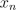be the robots position after n steps (sois a random variable) and let's define a new random variable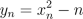. Also let's write E(-) for the average value of -. Then we have the equations: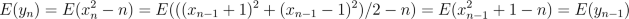Here the second equality comes from the fact that half the timeand the other half of the time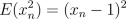. Since the average distance of the robot after 0 steps is 0, we see that the average distance of the robot from 0 is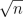.